PHY294H - Lecture 41

Spherical refracting surfaces

Last lecture, we saw that parallel rays incident upon a spherical mirror have a common focal point, provided that the angle of reflection is sufficiently small (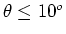). For concave mirrors the focal length is positive, for convex mirrors the focal length is negative. Parallel light rays incident upon a spherical refracting surface also have a common focal point. The relation between the focal length and the radius of the spherical surface is,(1)

Notice that in this case the focal length is larger than the radius of curvature and it depends on the refractive indices of the material inside the spherical surface (n2) and the material outside the spherical surface (n1).

Ray diagrams can again be used to show that an image is formed by a spherical refracting surface. The principal rays in this case are:

1. A parallel incoming ray is refracted through the focal point.

2. A ray which passes through the center of curvature is not refracted.

The relations between the image and source are now,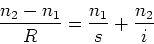(2)

and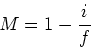(3)

The sign conventions for refracting surfaces are:

- The image distance is positive when it is on the opposite side to the source.

- The radius of curvature is taken to be positive when the center of curvature is on the opposite side to the approaching rays of light.

- The magnification is negative for inverted images.

Now we consider a more complex case, where we have two refracting surfaces. In this case we first treat the first refracting surface and find its image. Then we use that image as the source for the second refracting surface. This procedure sometimes leads to a new situation, namely cases where the image from the first refracting surface is on the real image side of the second refracting surface. This case can be treated with the formula above provided we introduce the sign convention:

- Object distances are negative if the object is on the real image side of the refracting surface.

With this definition and the rules for constructing rays given above, we can carry out calculations for a sequence of refracting surfaces. This enables us to derive the thin lens formulae given below.

Thin lenses

The thin lens laws are applications of the equations for a refracting surface. A thin lens is two refracting surfaces which are close to each other. If we place such a lens in air, the effect of the first refractive surface is to produce an image whose position is found from,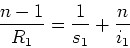(4)

which may be written as,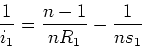(5)

This image acts as the object for the second refracting surface so that s2 = - i1The law relating the image and object distances for the second image is,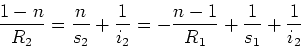(6)

This leads to the formula for a thin lens in air, the lens-maker's formula"(7)

The magnification is given by,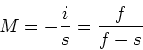(8)

Sign convention for R1 and R2

Again the rule is that the radii R1 or R2 are negative if the approaching rays of light are on the same side as the center of curvature.

Principle rays for thin lenses

- 1. An incoming ray parallel to the central axis of the lens is refracted to (or from) the focal point.

- 2. A ray that passes through the focal point is refracted to an outgoing ray which is parallel to the central axis(converging lens).

- 3. A ray passes through the center of the lens undeflected (this is a thin lens approximation).